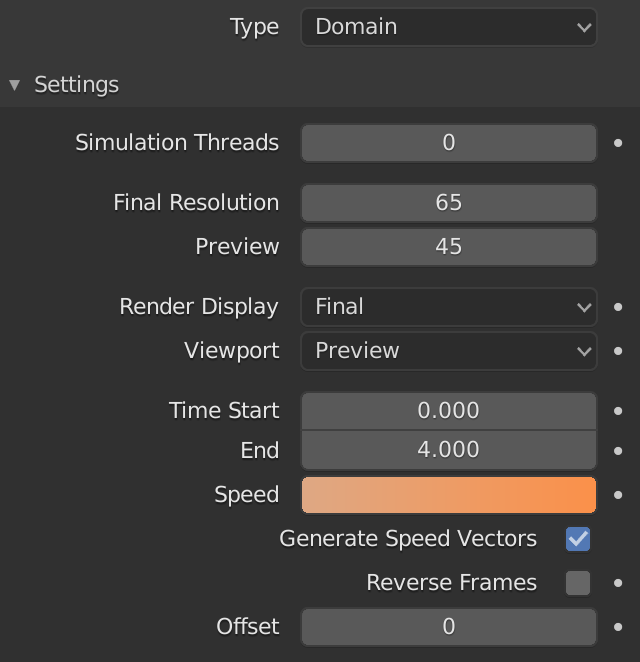# Speed

– Speed sets the “duration” of the simulation (NOT of the video!).

– E.g. for a 24 fps video, with 240 total frames (video duration 10 seconds):

– if Start Time=0 and End Time= 5 and Speed=1 we render 5 second of a fluid simulation in a 10 seconds video: (End Time – Start Time)*Speed=5

– if Start Time=0 and End Time= 5 and Speed=2 we render 10 second of a fluid simulation in a 10 seconds video: (End Time – Start Time)*Speed=10

– For this reason the second fluid animation is “faster” than the first (and “real”) one.

– In this example at 24 fps video a 5 seconds simulation is calculated at two different speeds: